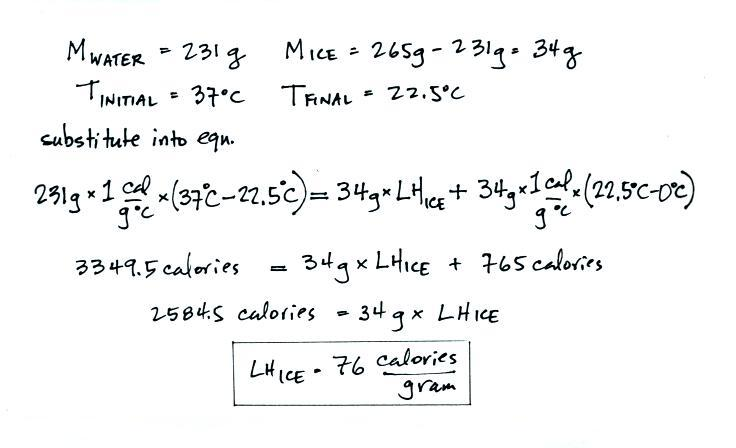Measuring the Latent Heat of Fusion of Ice

Object of the Experiment
Energy is required to change water from a solid to a liquid, i.e. to melt ice.  In this experiment you will try to measure the latent heat of fusion of ice (LHice), the energy needed (per gram) to melt ice.  The needed energy will come from a cup of warm water.  The amount of water and its temperature will be measured before adding some ice and then again after the ice has been melted.  These data will be used in an energy balance equation to determine LHice.

Conducting the Experiment
You will first need to obtain some ice.  Let the ice sit in a bowl at room temperature until you notice that it is starting to melt.  This will probably take 15 to 20 minutes.  In a freezer, ice is kept at a temperature well below freezing (typically near 0o F).  We need to allow the ice to sit outside the freezer long enough that is warms up to freezing (
0o C or 32o F).  The ice can't get warmer than freezing; once it reaches freezing it will start to melt.

Add 200 to 250 mL of warm tap water (
40o or less so that there isn't too big of a temperature difference between the water and air) to the styrofoam cup.  We keep the warm water in a styrofoam cup to minimize the loss of heat to the cooler surroundings.  Keep a record of the actual amount of water added to the cup.  Measure and record the temperature of the warm water just before you are ready to add some ice.

Add one or two ice cubes (or about 1/4 cup of crushed ice) to the warm water and stir gently with the thermometer until the ice is completely melted.  Try to be sure you just add ice and none of the water in the bowl from the melted ice.  Once the ice in the cup has melted, measure and record the water temperature.  If the temperature has dropped to near freezing or if you are unable to melt all of the ice, you will need to repeat the experiment.  You will need to use less ice or more water or both.

Use the graduated cylinder to measure the new volume of the water in the styrofoam cup.  This new volume will be larger than the initial volume because it contains the water from the melted ice.  Because water has a density of 1gram/mL (1 gram/cm3) there is a one to one relationship between a measurement of water volume in mL and the mass of the water in grams.  The difference in the starting and ending water volumes is the mass of the ice that was melted.

Repeat the experiment at least one more time using a different amount of water, a different amount of ice, and perhaps a different initial water temperature.

Data Analysis
The conditions at the start of an actual trial run of the experiment are shown below.  Tinitial is the temperature of the water water before the ice was added, Mwater is the mass of the warm water (note the mass is actually determined by measuring the intial volume of the water).  We assume that the ice has an initial temperature of
0o C because we let the ice sit at room temperature until it had started to melt.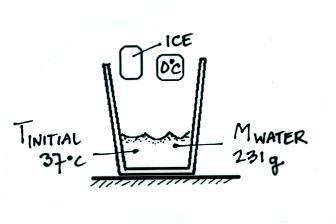In the figure below the ice has been added to the warm water and melted.  We are at the "midway" point of the experiment; the ice has turned from a solid to a liquid but its temperature hasn't changed, it is still 0oC.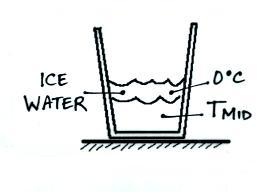The energy needed to melt the ice is: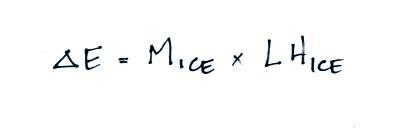This energy came from the warm water and cause the water to cool (from Tinitial to Tmid).  We could use the following equation to determine how much energy was taken from the water (we won't do the calculation because we don't know what Tmid is).Heat will continue to flow from the warm water into the 0o C ice water.  Energy will flow until all of the water has the same temperature, Tfinal.So the warm water will lose an additional amount of energy:The energy is used to warm the melted ice to Tfinal:Now we will combine all the separate terms.  Energy was removed from the water to first melt the ice and then to warm the ice water to Tfinal.  The toal energy lost by the warm water is:We perform the experiment in an insulated cup and we assume that all of the energy lost by the warm water was used to melt ice and warm up the ice water; no energy flowed from the cup into the cooler air in the room.  We'll add the 1st and 4th equations above together: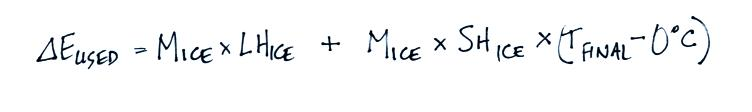We'll set the energy lost and energy used equations equal to each other.  This gives us an energy balance equation that can used to determine LHice: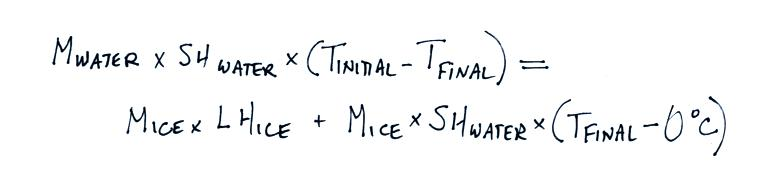The specific heat of water is 1 cal/gm oC.  You can plug this value together with your measured values for Mwater, Mice, Tinitial and Tfinal and solve for LHice.  An example calculation using the data shown in the figures above is given below.  The known value for the latent heat of fusion of ice is 80 calories/gram so the measured value below compares pretty well.